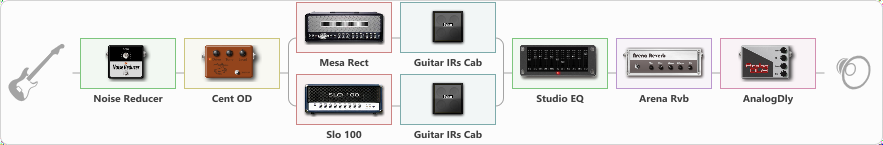Discussion in 'ToneLib-GFX presets' started by Dominique Sideleau, Aug 29, 2020.

Bridge pick-up

Effects chain:Effect: "Noise Reducer" (Dynamics / Filter), active - "yes"
{
"Sens" = 78
"Mode" = Soft
}

Effect: "Cent OD" (Overdrive / Distortion), active - "yes"
{
"Drive" = 38
"Tone" = 97
"Level" = 38
}

Effect: "Splitter" (Dynamics / Filter), active - "yes"
{
"A-Bypass" = Off
"A-Pan" = 0
"A-Level" = 55
"B-Bypass" = Off
"B-Pan" = 0
"B-Level" = 55

'A' branch:
{

Effect: "Mesa Rect" (Amp simulators), active - "yes"
{
"Gain" = 72
"Bass" = 50
"Middle" = 50
"Treble" = 76
"Presence" = 0
"Master" = 77
"Level (dB)" = 6
}

Effect: "Guitar IRs Cab" (Cabinets), active - "yes"
{
"Model" = Fender Deluxe Rev (1x12")
"Mic Position" = Middle
"Mic Distance" = Near
"Low Cut (Hz)" = 60
"Hi Cut (kHz)" = 20.0
"Mix" = 100
"Level (dB)" = 0
}
}
'B' branch:
{

Effect: "Slo 100" (Amp simulators), active - "yes"
{
"Gain" = 69
"Bass" = 50
"Middle" = 50
"Treble" = 72
"Presence" = 3
"Master" = 78
"Level (dB)" = 6
}

Effect: "Guitar IRs Cab" (Cabinets), active - "yes"
{
"Model" = Engl Pro (4x12")
"Mic Position" = Middle
"Mic Distance" = Near
"Low Cut (Hz)" = 60
"Hi Cut (kHz)" = 20.0
"Mix" = 100
"Level (dB)" = 0
}
}
}

Effect: "Studio EQ" (Dynamics / Filter), active - "yes"
{
"31 Hz" = 0
"62 Hz" = -4
"125 Hz" = -2
"250 Hz" = 3
"500 Hz" = 4
"1 kHz" = 1
"2 kHz" = 4
"4 kHz" = -5
"8 kHz" = 0
"16 kHz" = -3
"Level (dB)" = 2
}

Effect: "Arena Rvb" (Reverberation), active - "yes"
{
"Time" = 7.0
"PreDelay" = 40
"LoDamp" = 38
"HiDamp" = 20
"Mix" = 49
}

Effect: "AnalogDly" (Delay), active - "yes"
{
"Time" = 218
"Feedback" = 65
"Tone" = 45
"Mix" = 31
}

Note: You will need to download and install the ToneLib-GFX software to use the preset.